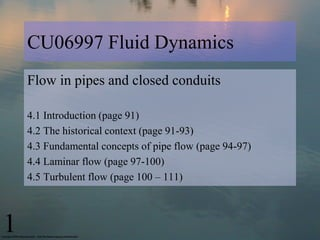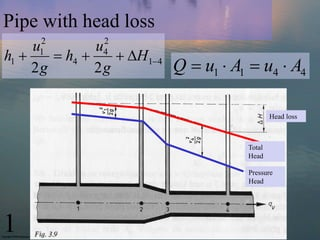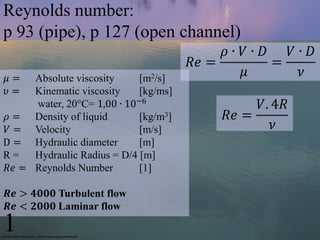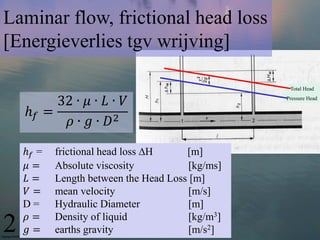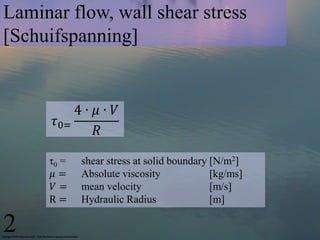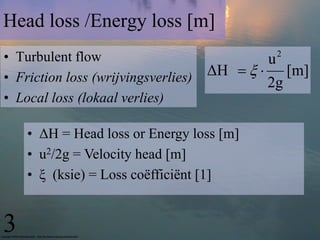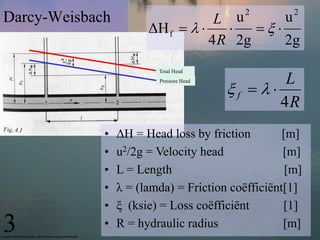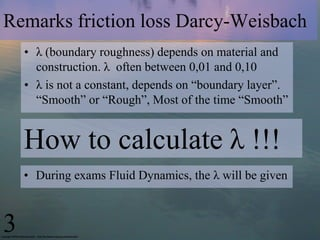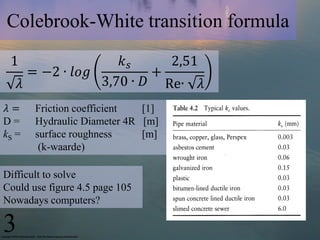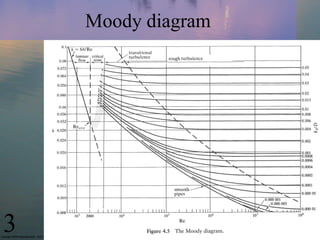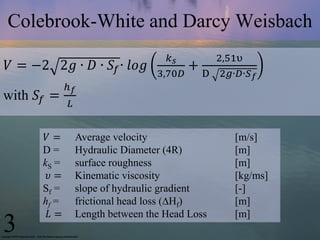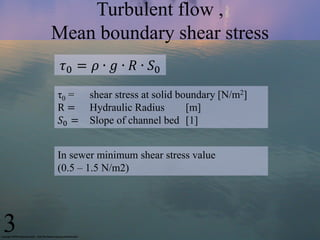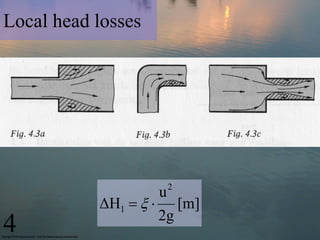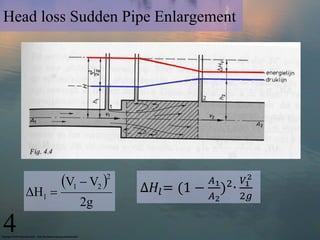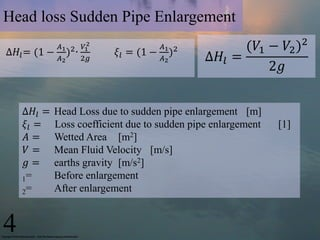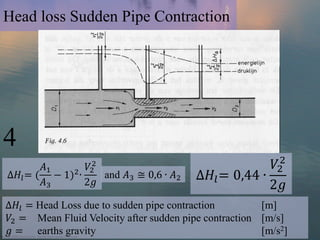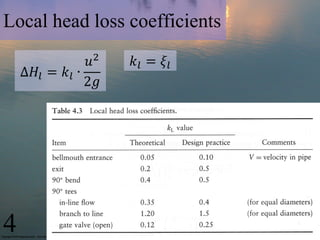1 of 17

### Cu06997 lecture 6_flow in pipes 1_2013

1. CU06997 Fluid Dynamics Flow in pipes and closed conduits 4.1 Introduction (page 91) 4.2 The historical context (page 91-93) 4.3 Fundamental concepts of pipe flow (page 94-97) 4.4 Laminar flow (page 97-100) 4.5 Turbulent flow (page 100 – 111) 1
2. Pipe with head loss 2 2 u u h1  1  h4  4  H14 2g 2g Q  u1  A1  u4  A4 Head loss Total Head Pressure Head 1
3. Reynolds number: p 93 (pipe), p 127 (open channel) 𝜌∙ 𝑉∙ 𝐷 𝑉∙ 𝐷 𝑅𝑒 = = 𝜇= Absolute viscosity [m2/s] 𝜇 𝜈 𝜐= Kinematic viscosity [kg/ms] water, 20°C= 1,00 ∙ 10−6 𝑉. 4𝑅 𝜌 = Density of liquid [kg/m3] 𝑅𝑒 = 𝑉 = Velocity [m/s] 𝜈 D = Hydraulic diameter [m] R= Hydraulic Radius = D/4 [m] 𝑅𝑒 = Reynolds Number  𝑹𝒆 > 𝟒𝟎𝟎𝟎 Turbulent flow 𝑹𝒆 < 𝟐𝟎𝟎𝟎 Laminar flow 1
4. Laminar flow, frictional head loss [Energieverlies tgv wrijving] Total Head 32 ∙ 𝜇 ∙ 𝐿 ∙ 𝑉 Pressure Head ℎ𝑓 = 𝜌 ∙ 𝑔 ∙ 𝐷2 ℎ𝑓 = frictional head loss ∆H [m] 𝜇= Absolute viscosity [kg/ms] 𝐿= Length between the Head Loss [m] 𝑉= mean velocity [m/s] D= Hydraulic Diameter [m] 𝜌= Density of liquid [kg/m3] 2 𝑔= earths gravity [m/s2]
5. Laminar flow, wall shear stress [Schuifspanning] 4∙ 𝜇∙ 𝑉 𝜏0= 𝑅 τ0 = shear stress at solid boundary [N/m2] 𝜇= Absolute viscosity [kg/ms] 𝑉= mean velocity [m/s] R= Hydraulic Radius [m] 2
6. Head loss /Energy loss [m] • Turbulent flow u 2 • Friction loss (wrijvingsverlies) ΔΗ    [m] 2g • Local loss (lokaal verlies) • ΔH = Head loss or Energy loss [m] • u2/2g = Velocity head [m] • ξ (ksie) = Loss coëfficiënt  3
7. 2 2 Darcy-Weisbach L u u ΔΗ f       4 R 2g 2g Total Head L f   Pressure Head 4R • ΔH = Head loss by friction [m] • u2/2g = Velocity head [m] • L = Length [m] • λ = (lamda) = Friction coëfficiënt • ξ (ksie) = Loss coëfficiënt  3 • R = hydraulic radius [m]
8. Remarks friction loss Darcy-Weisbach • λ (boundary roughness) depends on material and construction. λ often between 0,01 and 0,10 • λ is not a constant, depends on “boundary layer”. “Smooth” or “Rough”, Most of the time “Smooth” How to calculate λ !!! • During exams Fluid Dynamics, the λ will be given 3
9. Colebrook-White transition formula 1 𝑘𝑠 2,51 = −2 ∙ 𝑙𝑜𝑔 + 𝜆 3,70 ∙ 𝐷 Re∙ 𝜆 𝜆= Friction coefficient  D= Hydraulic Diameter 4R [m] kS = surface roughness [m] (k-waarde) Difficult to solve Could use figure 4.5 page 105 Nowadays computers? 3
10. Moody diagram 3
11. Colebrook-White and Darcy Weisbach 𝑘𝑠 2,51υ 𝑉 = −2 2𝑔 ∙ 𝐷 ∙ 𝑆 𝑓 ∙ 𝑙𝑜𝑔 + 3,70𝐷 D 2𝑔∙𝐷∙𝑆 𝑓 ℎ𝑓 with 𝑆 𝑓 = 𝐿 𝑉= Average velocity [m/s] D= Hydraulic Diameter (4R) [m] kS = surface roughness [m] 𝜐= Kinematic viscosity [kg/ms] Sf = slope of hydraulic gradient [-] hf = frictional head loss (∆Hf) [m] 𝐿= Length between the Head Loss [m] 3
12. Turbulent flow , Mean boundary shear stress 𝜏0 = 𝜌 ∙ 𝑔 ∙ 𝑅 ∙ 𝑆0 τ0 = shear stress at solid boundary [N/m2] R= Hydraulic Radius [m] 𝑆0 = Slope of channel bed  In sewer minimum shear stress value (0.5 – 1.5 N/m2) 3
13. Local head losses 2 u ΔΗ l    [m] 2g 4
14. Head loss Sudden Pipe Enlargement V1  V2  2 ∆𝐻 𝑙 = (1 − 𝐴1 2 𝑉1 ) ∙ 2 ΔΗ l  𝐴2 2𝑔 2g 4
15. Head loss Sudden Pipe Enlargement ∆𝐻 𝑙 = (1 − 𝐴1 2 𝑉1 ) ∙ 2 𝜉 𝑙 = (1 − 𝐴1 2 ) (𝑉1 − 𝑉2 )2 𝐴2 2𝑔 𝐴2 ∆𝐻 𝑙 = 2𝑔 ∆𝐻 𝑙 = Head Loss due to sudden pipe enlargement [m] 𝜉𝑙 = Loss coefficient due to sudden pipe enlargement  𝐴= Wetted Area [m2] 𝑉= Mean Fluid Velocity [m/s] 𝑔= earths gravity [m/s2] 1= Before enlargement 2= After enlargement 4
16. Head loss Sudden Pipe Contraction 4 2 𝐴1 2 𝑉2 𝑉2 ∆𝐻 𝑙 = ( − 1)2 ∙ 𝐴3 2𝑔 and 𝐴3 ≅ 0,6 ∙ 𝐴2 ∆𝐻 𝑙 = 0,44 ∙ 2𝑔 ∆𝐻 𝑙 = Head Loss due to sudden pipe contraction [m] 𝑉2 = Mean Fluid Velocity after sudden pipe contraction [m/s] 𝑔 = earths gravity [m/s2]
17. Local head loss coefficients 𝑢2 𝑘 𝑙 = 𝜉𝑙 ∆𝐻 𝑙 = 𝑘 𝑙 ∙ 2𝑔 4